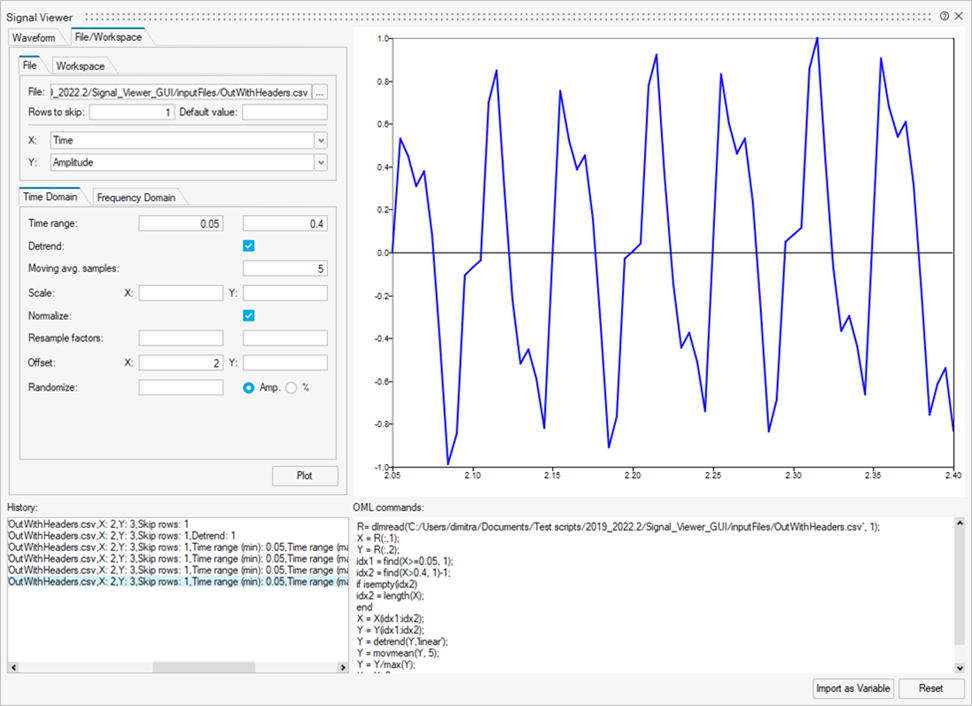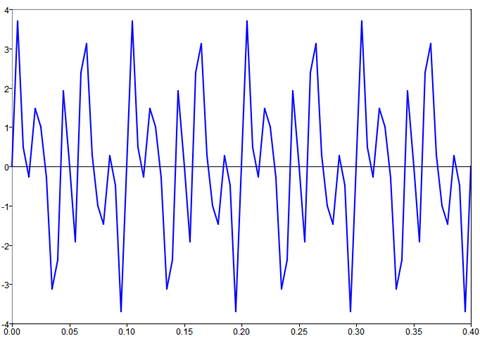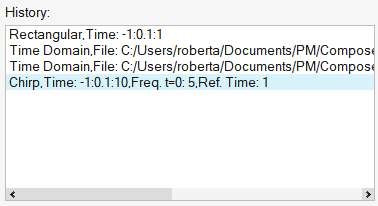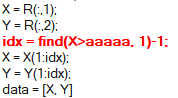# Signal Viewer

Use the Signal Viewer to import and treat (basic) signals in both time and frequency domains, as well as generate signals based on the most common waveforms such as step, chirp and sawtooth.The Signal Viewer offers the following functionality:

## Plotting

The utility plots information as defined through the waveform or file, and signal processing options:## History

The History dialog displays the Signal Viewer events in your session. You can view or reapply any event by double-clicking it.## OML Command Generation

The OML Commands window autogenerates the OML syntax for producing the same output that is displayed in the Plot window. The same defined actions and visual information produced are printed in this area for reuse in an OML script.

When a certain input is incorrect and the application is unable to generate the plot, the problematic command is written in red in the Output area:The context menu of the OML Commands menu offers additional options aside from the standard Copy and Select All options:
• Run Selection: Evaluates the selected commands.
• Copy to Editor: Copies the selected commands in the active OML script editor.

If an OML script editor does not exist, or if one exists but is not active, a new OML script editor is created and the commands are pasted.

Note: OML offers additional functionality that you can leverage if your project requires further or advanced data treatment.

## Import Data as a Variable

Use the Import as Variable button to import the visualized data as a variable. The default variable name is data. If the variable name already exists in your OML workspace, a dialog prompts you to rename or overwrite the variable. If you select Rename, a new dialog prompts you to enter a new variable name.

## Reset

The Reset button clears the Signal Viewer interface and resets it to its initial state.

## Waveform Tab

Create a signal based on a standard waveform, such as chirp and sawtooth.

The input parameters are divided in mandatory and optional arguments. The mandatory ones have suggested values to give hints on the expected input syntax that must be used.
Waveform Option Description
Chirp Swept-frequency wave, in which the frequency increases or decreases with time. If omitted, the optional parameters are:
• Reference time: 1 sec
• Frequency at reference time: 100 Hz
• Shape: linear
• Phase: 0
Gauss Gaussian convolution window. If omitted, the optional parameters are:
• Center frequency: 1000 Hz
• Fractional bandwidth: 0.5
Random Normally distributed random signal.
Rectangular Rectangular wave. If omitted, the optional parameters are:
• Width: 1
Sawtooth Sawtooth signal. If omitted, the optional parameters are set as:
• Width: 1
Sinusoidal Standard sine wave. If omitted, the optional parameters are:
Square Square wave. If omitted, the optional parameters are:
• Duty: 50
Triangular
• Width: 1
• Skew: 0

## File/Workspace Tab

Import files and select variables.

### File

Import files that are supported in the dlmread command, such as delimited files.

File import options include:

• Rows to skip: The number of rows from the beginning of the file that dlmread does not read. This is useful when a file contains multiple lines of headers before the numeric data. The Signal Viewer tries to determine the number of rows to skip automatically when a file is selected.
• Default value: The value to be used in the output for empty or non-numeric values of the input file. If left empty, the value is set to 0.

If the file contains a header in its first line, the different columns will be named after this header. If not, the columns are simply called Column 1, Column 2 and so on.

If the Rows to skip or Default value values have changed, when you click the Plot button, the file is reloaded. If the file has changes since the last time it was loaded, you are prompted to select whether to reload the file or use the data already loaded in the Signal Viewer.

Every time the file is loaded in the Signal Viewer, an information label is shown in the lower left corner of the window.

### Workspace

Select variables from the workspace. Only vectors and matrices are accepted. If the variable is a matrix, the column can also be selected.

### Time Domain

Applies the following actions in the time domain:

Time Domain Option Description
Time Range Cuts the signal from a lower to an upper value.
Detrend Detrends the signal using the linear method, that is, removing the best fit trend.
Moving Avg. Samples Applies a moving average filter based on a certain number of input samples.
Scale Scales the signal in X and/or Y by a certain factor.
Normalize Normalizes the signal.
Offset Resamples the signal by a rational factor using a polyphase Finite Impulse Response (FIR) filter.
Randomize Applies white noise in the signal either using a Signal-to-Noise (S/N) ratio or a certain percentage of noise:
• S/N ratio: procedure similar to
``````noise_power = (N_S / 100) * signal_power;
sigma = sqrt(noise_power / length(signal));
noise = normrnd(0, sigma, size(signal));
signal_mod = signal + noise;``````
• Percentage: two new signals are originated, one with the original signal summed up with the given percentage of it and another with the original signal subtracted from the given percentage of it. The new upper and lower signal are subtracted from each other, multiplied by a random signal with the same size and summed up with the lower signal.
``````noise_power = 10^(-1/(20 *N_S)) * signal_power;
ran_upper = signal + ((N_S/100)*signal);
ran_lower = signal - ((N_S/100)*signal);
random_gen = rand(length(signal),1);
signal_mod = (ran_upper - ran_lower) .* random_gen + ran_lower;``````

The actions are performed in the order that they appear, for example: Cut the signal > Detrend it > Apply moving average filter > Scale it > Normalize it > Resample it > Offset it > Randomize it

### Frequency Domain

Applies the following actions in frequency domain:

Frequency Domain Option Description
FFT Size Computes Fast Fourier Transform (FFT) based on the size input. If the size input is not given, the signal length is used by default. The FFT is computed, normalized and folded to generate a single-sided output.
Frequency Range Applies a frequency-domain filter:
• Only first (lower) value is given: highpass filter.
• Only second (upper) value is given: lowpass filter.
• Both values are given: bandpass filter.
Compute PSD
• Checkbox to compute the Power Spectral Density (PSD) of the signal.
Window Select the PSD checkbox to apply a windowing method to the signal to reduce spectral leakage. If the checkbox is not selected, the PSD is calculated without windowing. The options are:
• None
• Blackman
• Dolph-Chebyshev
• Hann
• Hamming
• Kaiser
• Parzen
• Welch
Window overlap Number of samples that are simultaneously in different windows. To use window overlap, select the PSD checkbox and enter a windowing method other than None. If a method is not provided, the Power Spectral density is calculated without windowing.